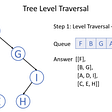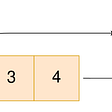# DP with Monotonic queue: 1696 Jump Game VI

## DP look forward TLE (O(n*k))

`class Solution {public:    int maxResult(vector<int>& nums, int k) {        const int n = nums.size();        int ret = 0;        vector<int> dp(n, INT_MIN);        dp = nums;        for (int i = 0; i < n; ++i) {            for (int j = 1; j <= k; ++j) {                if (i+j < n) {                    dp[i+j] = max(dp[i+j], dp[i] + nums[i+j]);                }            }        }        return dp[n-1];         }};`

## DP look backward TLE (O(n*k))

`class Solution {public:    int maxResult(vector<int>& nums, int k) {        const int n = nums.size();        int dp[n];        dp = nums;        for (int i = 1; i < n; ++i) {            int thisMax = dp[i-1];            for (int j = k; j > 1; --j) {                if (i - j >= 0) thisMax = max(thisMax, dp[i-j]);            }            dp[i] = thisMax + nums[i];        }        return dp[n-1];    }};`

## Look backward DP with multiple set solution (O(n log k))

`class Solution {public:    int maxResult(vector<int>& nums, int k) {        const int n = nums.size();        int dp[n];        dp = nums;        multiset<int> ms;        ms.insert(dp);        for (int i = 1; i < n; ++i) {            // remove outdated one            if (i - k -1 >= 0) ms.erase(dp[i-k - 1]);            dp[i] = *ms.rbegin() + nums[i];            // add new one            ms.insert(dp[i]);        }        return dp[n-1];    }};`

## Look backward DP with Monotonic queue O(n) solution

`class Solution {public:    int maxResult(vector<int>& nums, int k) {        int n = nums.size();        vector<int> f(n);        f = nums;        deque<int> q = {0};        for (int i = 1; i < n; ++i) {            while (i - q.front() > k) {                q.pop_front();            }            f[i] = f[q.front()] + nums[i];            while (!q.empty() && f[i] >= f[q.back()]) {                q.pop_back();            }            q.push_back(i);        }        return f[n - 1];    }};`
`class Solution {public:    int maxResult(vector<int>& nums, int k) {        const int n = nums.size();        int dp[n];        dp = nums;        // Elements in the DQ is in increadig order.        deque<int> DQ{0};        for (int i = 1; i < n; ++i) {            // pop the invalid elements from back,             // then the last element will be the largest one in the sliding window.            while(!DQ.empty() && DQ.back() < i - k) DQ.pop_back();            // the following step is unnecessary (add it will also be fine),             // as the above step can garuantee that the used one is valid.            // while(!DQ.empty() && DQ.front() < i - k) DQ.pop_front();            // harvest the largest one            dp[i] = dp[DQ.back()] + nums[i];            // push the current one into the DQ.            // if the current one is larger than any old value, pop the old one,            // use this fresh one as the fresh one can only be better than old one (unless old one is larger than the current one).            while (!DQ.empty() && dp[i] >= dp[DQ.front()]) DQ.pop_front();            DQ.push_front(i);        }        return dp[n-1];    }};`

--

--

--

## More from Jimmy Shen

Data Scientist/MLE/SWE @takemobi

Love podcasts or audiobooks? Learn on the go with our new app.

## ClayStack Victoria Goes Live Today!## Pandemic Juniors## How ZettaBytes(1000000000000 GB) of data is stored permanently with low cost and High I/O?## Immutable Deployment Pattern for ForgeRock Access Management (AM) Configuration without File Based…## Learning to Code — Part 9b: Inheritance & Polymorphism## PLUTOS NETWORK LAUNCHED A USER DASHBOARD ALONGSIDE A GAME CALLED PLUTBUTTON## Jimmy Shen

Data Scientist/MLE/SWE @takemobi

## Designing an Engineering Take Home Assignment — Part A## 5/4 Binary Search Tree## 2022. Convert 1D Array Into 2D Array — LeetCode(Python)